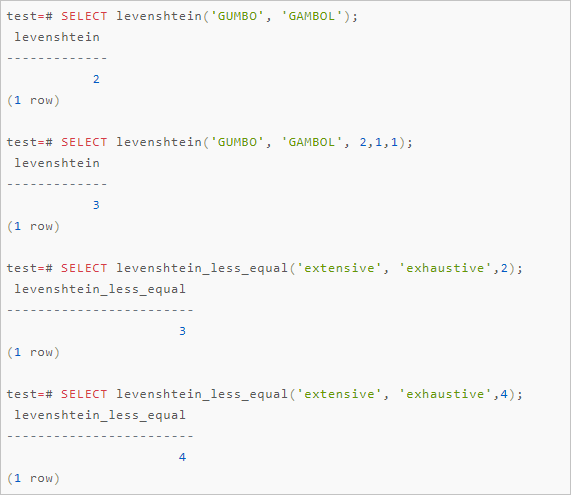ApsaraDB RDS for PostgreSQL provides the fuzzystrmatch plug-in. This plug-in supports the Soundex, Levenshtein, Metaphone, and Double Metaphone algorithms. You can use these algorithms to calculate the similarity and distance between strings.

## Soundex

The Soundex algorithm converts similar-sounding words into the same code. However, this algorithm is unsuitable for non-English words.

The Soundex algorithm provides the following functions:

``````soundex(text) returns text
difference(text, text) returns int``````
• The soundex function converts a string into its Soundex code, such as A550.
• The difference function converts two strings into their Soundex codes. Then, the difference function reports the number of code matching positions between the two strings. A Soundex code consists of four characters. Therefore, the number of code matching positions ranges from 0 to 4. The value 0 indicates a zero match, and the value 4 indicates an exact match.

Examples:

``````SELECT soundex('hello world!') ;
SELECT soundex('Anne'), soundex('Andrew'), difference('Anne', 'Andrew');
SELECT soundex('Anne'), soundex('Margaret'), difference('Anne', 'Margaret');

CREATE TABLE s (nm text);
INSERT INTO s VALUES ('john');
INSERT INTO s VALUES ('joan');
INSERT INTO s VALUES ('wobbly');
INSERT INTO s VALUES ('jack');

SELECT * FROM s WHERE soundex(nm) = soundex('john');
SELECT * FROM s WHERE difference(s.nm, 'john') > 2;``````

## Levenshtein

The Levenshtein algorithm calculates the Levenshtein distance between two strings.

The Levenshtein algorithm provides the following functions:

``````levenshtein(text source, text target, int ins_cost, int del_cost, int sub_cost) returns int
levenshtein(text source, text target) returns int
levenshtein_less_equal(text source, text target, int ins_cost, int del_cost, int sub_cost, int max_d) returns int
levenshtein_less_equal(text source, text target, int max_d) returns int``````

The following table describes the parameters that you must configure in the preceding functions.

Parameter Description
source The first string to compare. The string cannot be empty and can contain up to 255 characters in length.
target The second string to compare. The string cannot be empty and can contain up to 255 characters in length.
ins_cost The overhead that is required to insert characters.
del_cost The overhead that is required to delete characters.
sub_cost The overhead that is required to replace characters.
max_d The maximum Levenshtein distance that is allowed between the two specified strings.
Note The levenshtein_less_equal function is an accelerated version of the levenshtein function. It is used only to calculate a short Levenshtein distance:
• If the actual distance is less than or equal to the value of the max_d parameter, the levenshtein_less_equal function returns the exact distance that is calculated.
• If the actual distance is greater than the value of the max_d parameter, the levenshtein_less_equal function returns a random distance that is greater than the value of the max_d parameter.
• If the value of the max_d parameter is negative, the levenshtein_less_equal and levenshtein functions return the same distance.

Examples:

``````SELECT levenshtein('GUMBO', 'GAMBOL');
SELECT levenshtein('GUMBO', 'GAMBOL', 2,1,1);
SELECT levenshtein_less_equal('extensive', 'exhaustive',2);
SELECT levenshtein_less_equal('extensive', 'exhaustive',4);``````## Metaphone

The Metaphone algorithm works in the same way as the Soundex algorithm. The Metaphone algorithm constructs a representative code for each specified string. If two strings have the same representative code, the Metaphone algorithm considers them to be similar.

The Metaphone algorithm provides the following functions:

``metaphone(text source, int max_output_length) returns text``

The following table describes the parameters that you must configure in the preceding functions.

Parameter Description
source A string that is not empty. The string can contain up to 255 characters in length.
max_output_length The maximum length of the Metaphone code that can be returned. If the Metaphone code exceeds the maximum length, the Metaphone algorithm truncates the Metaphone code to the maximum length.

Example:

``SELECT metaphone('GUMBO', 4);``

## Double Metaphone

The Double Metaphone algorithm obtains two similar-sounding codes for a specified string. These codes include a primary code and a secondary code. In most cases, the two codes are the same. They may be slightly different when you specify a non-English word. The difference varies based on the pronunciation.

The Double Metaphone algorithm provides the following functions:

``````dmetaphone(text source) returns text
dmetaphone_alt(text source) returns text``````

Examples:

``````select dmetaphone('gumbo');
select dmetaphone_alt('gumbo');``````